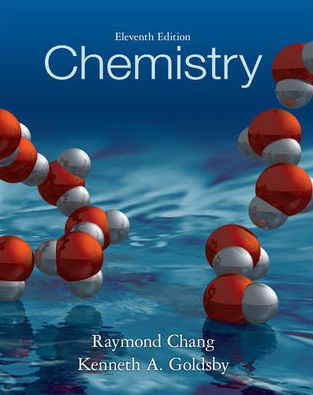×
Get Full Access to Chemistry - 11 Edition - Chapter 7 - Problem 76p
Get Full Access to Chemistry - 11 Edition - Chapter 7 - Problem 76p

×

# Answer: The ground-state electron configurations listedISBN: 9780073402680 118

## Solution for problem 76P Chapter 7

Chemistry | 11th Edition

• Textbook Solutions
• 2901 Step-by-step solutions solved by professors and subject experts
• Get 24/7 help from StudySoup virtual teaching assistantsChemistry | 11th Edition

4 5 1 252 Reviews
29
1
Problem 76P

The ground-state electron configurations listed here are incorrect. Explain what mistakes have been made in each and write the correct electron configurations.

AI: $$1 s^{2} 2 s^{2} 2 p^{4} 3 s^{2} 3 p^{3}$$

B: $$1 s^{2} 2 s^{2} 2 p^{5}$$

F: $$1 s^{2} 2 s^{2} 2 p^{6}$$

Step-by-Step Solution:

Step 1 of 4

Here we have to correct the ground state electronic configuration of the given atoms.

Step 2 of 4

Step 3 of 4

## Discover and learn what students are asking

Calculus: Early Transcendental Functions : The Natural Logarithmic Function: Integration
?In Exercises 1-26, find the indefinite integral. $$\int \frac{10}{x} d x$$

Unlock Textbook Solution

Enter your email below to unlock your verified solution to:

Answer: The ground-state electron configurations listed